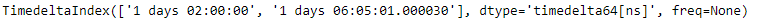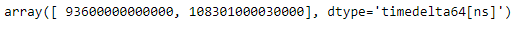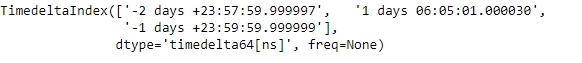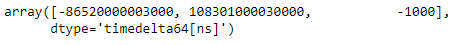# Python | Pandas TimedeltaIndex.base

• Last Updated : 29 Dec, 2018

Python is a great language for doing data analysis, primarily because of the fantastic ecosystem of data-centric python packages. Pandas is one of those packages and makes importing and analyzing data much easier.

Pandas` TimedeltaIndex.base` attribute return the base object if the memory of the underlying data is shared by more than one objects.

Syntax: TimedeltaIndex.base

Return : array

Example #1: Use `TimedeltaIndex.base` attribute to find out the base address of the TimedeltaIndex object.

 `# importing pandas as pd``import` `pandas as pd`` ` `# Create the TimedeltaIndex object``tidx ``=` `pd.TimedeltaIndex(data ``=``[``'1 days 02:00:00'``, ``'1 days 06:05:01.000030'``])`` ` `# Print the TimedeltaIndex``print``(tidx)`` ` `# Create a copy``tidx_copy ``=` `tidx`

Output :Now we want to find out the base address of these two objects

 `# Print the base address of original object``tidx.base`` ` `# Print the base address of the copied object``tidx_copy.base`

Output :As we can see in the output, the `TimedeltaIndex.base` attribute has returned the same base address for both the objects.

Example #2: Use `TimedeltaIndex.base` attribute to find out the base address of the TimedeltaIndex object.

 `# importing pandas as pd``import` `pandas as pd`` ` `# Create the TimedeltaIndex object``tidx ``=` `pd.TimedeltaIndex(data ``=``[``'-1 days 2 min 3us'``, ``'1 days 06:05:01.000030'``,``                                                 ``'-1 days + 23:59:59.999999'``])`` ` `# Print the TimedeltaIndex``print``(tidx)`` ` `# Create a copy``tidx_copy ``=` `tidx`

Output :Now we want to find out the base address of these two objects

 `# Print the base address of original object``tidx.base`` ` `# Print the base address of the copied object``tidx_copy.base`

Output :As we can see in the output, the `TimedeltaIndex.base` attribute has returned the same base address for both the objects.

My Personal Notes arrow_drop_up